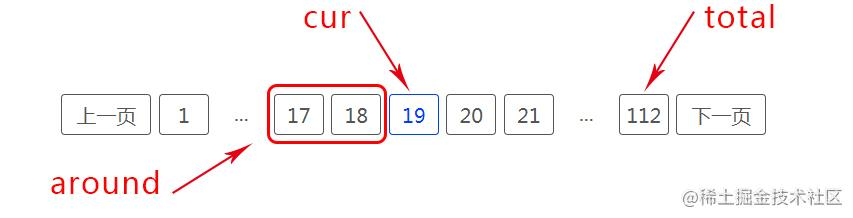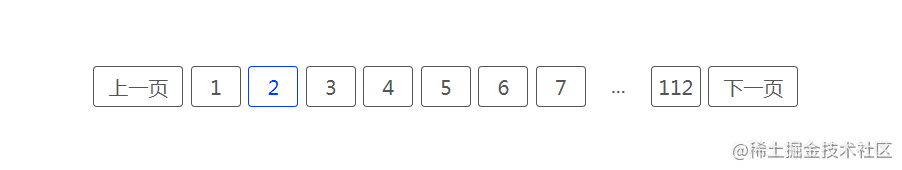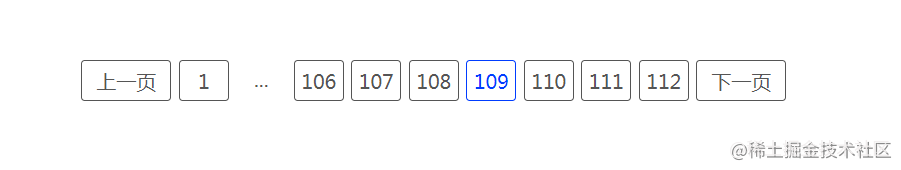# 十五分钟--分页逻辑--包学包会

### 分页组件的构成

`````` 《上一页》《下一页》 2 个 ： 2
《首页》《尾页》 2 个 ： 2
省略号 2 个 ： 2
当前页 1 个 ： 1
当前页左右各2个 ：2 * 2### 省略号的作用

1、只出现在后面2、只出现在前面a.先确定只出现在后面的情况（前面即将出现省略号），首先确定什么时候前面刚好出现省略号？根据刚刚得出的省略号的作用，也就是说它最少代替两个元素。所以我们就取这个最小值2，此时是在前面刚刚出现省略号，省略号代表的越多则说明cur越往后。

b.同样的逻辑，我们还可以得出只在前面出现，后面不会出现省略号的一个临界位置：`endPosition` = 尾页(total) - 省略号最小值(2) - cur 右边的值(around) - 1。当cur > endPosition时只在前面出现省略号。

ok，逻辑分析已经完成了95%，还剩下一种两边都出现省略号的情况，我们不用分析了，除去刚才的两种情况就是它了，也就是当 `startPosition <= cur && cur <= endPosition`

### 其他的位置怎么显示

``````/**
*
* @param {Number} total
* @param {Number} cur
* @param {Numbre} around
*/
const makePage = (total,cur,around) => {
let result = [];
let baseCount = around * 2 + 1 + 2 + 2 + 2; //总共元素个数
let surplus = baseCount - 4; //只出现一个省略号 剩余元素个数
let startPosition = 1 + 2 + around + 1;//前面出现省略号的临界点
let endPosition = total - 2 - around - 1;//后面出现省略号的临界点

if(total <= baseCount - 2){ //全部显示 不出现省略号
result =  Array.from({length: total}, (v, i) => i + 1);
}else{ //需要出现省略号
if(cur < startPosition){ //1.只有后面出现省略号
result = [...Array.from({length: surplus}, (v, i) => i + 1),"...",total]
}else if(cur > endPosition) { //2.只有前边出现省略号
result = [1,'...',...Array.from({length: surplus}, (v, i) => total - surplus + i + 1)]
}else{ //3.两边都有省略号
result = [1,'...',...Array.from({length: around * 2 + 1}, (v, i) => cur - around + i),'...',total]
}
}

return result
}
makePage(8,2,2);//[1, 2, 3, 4, 5, 6, 7, 8]
makePage(20,3,2);//[1, 2, 3, 4, 5, 6, 7, "...", 20]
makePage(20,10,2);//[1, "...", 8, 9, 10, 11, 12, "...", 20]
makePage(20,19,2);//[1, "...", 14, 15, 16, 17, 18, 19, 20]